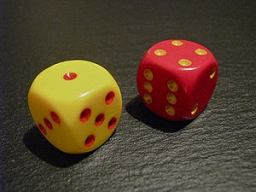# Probability 8376

Three players roll the dice. They can start the game when a six is ​​rolled. Everyone rolls 1 time.
a) What is the probability that exactly one starts in the first round?
b) What is the probability that at least two start?

p1 =  0.3472
p2 =  0.0741

### Step-by-step explanation:Did you find an error or inaccuracy? Feel free to write us. Thank you!

Tips for related online calculators